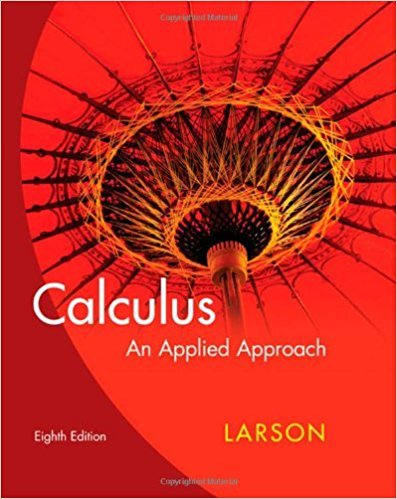×
×

# Solutions for Chapter 6: Techniques of Integration## Full solutions for Calculus: An Applied Approach | 8th Edition

ISBN: 9780618958252Solutions for Chapter 6: Techniques of Integration

Solutions for Chapter 6
4 5 0 329 Reviews
30
4
##### ISBN: 9780618958252

This textbook survival guide was created for the textbook: Calculus: An Applied Approach , edition: 8. This expansive textbook survival guide covers the following chapters and their solutions. Chapter 6: Techniques of Integration includes 60 full step-by-step solutions. Since 60 problems in chapter 6: Techniques of Integration have been answered, more than 23954 students have viewed full step-by-step solutions from this chapter. Calculus: An Applied Approach was written by and is associated to the ISBN: 9780618958252.

Key Calculus Terms and definitions covered in this textbook
• Acceleration due to gravity

g ? 32 ft/sec2 ? 9.8 m/sec

P(A or B) = P(A) + P(B) - P(A and B). If A and B are mutually exclusive events, then P(A or B) = P(A) + P(B)

• Anchor

See Mathematical induction.

• Coefficient

The real number multiplied by the variable(s) in a polynomial term

• Complex plane

A coordinate plane used to represent the complex numbers. The x-axis of the complex plane is called the real axis and the y-axis is the imaginary axis

• Cone

See Right circular cone.

• Coterminal angles

Two angles having the same initial side and the same terminal side

• Direct variation

See Power function.

• Distance (in Cartesian space)

The distance d(P, Q) between and P(x, y, z) and Q(x, y, z) or d(P, Q) ((x ) - x 2)2 + (y1 - y2)2 + (z 1 - z 2)2

• Inverse reflection principle

If the graph of a relation is reflected across the line y = x , the graph of the inverse relation results.

• Law of sines

sin A a = sin B b = sin C c

• Opens upward or downward

A parabola y = ax 2 + bx + c opens upward if a > 0 and opens downward if a < 0.

• Pascal’s triangle

A number pattern in which row n (beginning with n = 02) consists of the coefficients of the expanded form of (a+b)n.

• Placebo

In an experimental study, an inactive treatment that is equivalent to the active treatment in every respect except for the factor about which an inference is to be made. Subjects in a blind experiment do not know if they have been given the active treatment or the placebo.

• Slant asymptote

An end behavior asymptote that is a slant line

• Solution of an equation or inequality

A value of the variable (or values of the variables) for which the equation or inequality is true

• Sphere

A set of points in Cartesian space equally distant from a fixed point called the center.

• Synthetic division

A procedure used to divide a polynomial by a linear factor, x - a

• Vertical component

See Component form of a vector.

• Wrapping function

The function that associates points on the unit circle with points on the real number line

×